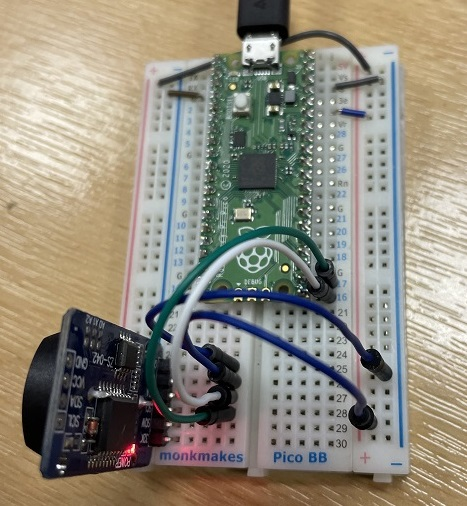# Raspberry Pi Pico RTC DS3231

The DS3231 is another RTC that works in a very similar way to the DS1338. Writing the library for this just took a few tweaks of the one I had previously written. The boards for these vary in price but you can pick some of them up very cheaply. The one I used for this circuit is shown below, cost about £3 and came with a rechargeable battery.I connected this as I did for the DS1338. I used GP16 for SDA, GP17 for SCL. VCC and GND were connected to 3V and ground.The class library needs a few alterations to work. The registers are at the same addresses but there are slight differences in how the values are stored. Save this to the Pico as ds3231.py. Remember to set the time the first time you use it.

```from machine import Pin, I2C

def bcd2bin(value):
return (value or 0) - 6 * ((value or 0) >> 4)

def bin2bcd(value):
return (value or 0) + 6 * ((value or 0) // 10)

class rtc:
def __init__(self, d, c):
self.i2c=I2C(0,sda=Pin(d), scl=Pin(c))

def get_time(self):
s = bcd2bin(buf)
m = bcd2bin(buf)
if buf & 0x40:
h = bcd2bin(buf & 0x1F)
if buf & 0x20:
h += 12
else:
h = bcd2bin(buf)
w = bcd2bin(buf)
dd = bcd2bin(buf)
mm = bcd2bin(buf & 0x1F)
yy = bcd2bin(buf) + 2000
return [s,m,h,w,dd,mm,yy]

def set_time(self,s,m,h,w,dd,mm,yy):
tm = bytes([bin2bcd(i) for i in [s,m,h,w,dd,mm,yy-2000]])
self.i2c.writeto_mem(int(0x68), int(0),tm)

```

Test with the following,

```from ds3231 import rtc
from time import sleep

myclock = rtc(16, 17)

# s,m,h,w,dd,mm,yy
#myclock.set_time(0,19,10,4,3,2,2022)

while True:
t = myclock.get_time()
hr = str(t)
mins = str(t)
secs = str(t)
if len(hr)<2: hr = "0" + hr
if len(mins)<2: mins = "0" + mins
if len(secs)<2: secs = "0" + secs
mytime = str(hr) + ":" + str(mins) + ":" + str(secs)
print(mytime)
sleep(1)
```